# Integral invariant

(diff) ← Older revision | Latest revision (diff) | Newer revision → (diff)

of degree (order), of a smooth dynamical system

An absolute integral invariant is an exterior differential formof degreethat is transformed into itself by the transformations generated by this system.

A relative integral invariant is an exterior differential formof degreewhose exterior differential is an absolute integral invariant (of degree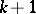).

One usually speaks of integral invariants of a flow (continuous-time dynamical system)defined by a system of ordinary linear differential equations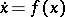, whereis a smooth vector field given on some domain in a Euclidean space (or on a manifold). In coordinates (local coordinates in the case of a manifold) this system has the form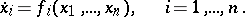(1)

An important example of an integral invariant is a volume form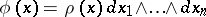(whereis a positive locally integrable (often even continuous or smooth) function in the coordinates). For smooththis form is an absolute invariant of (1) if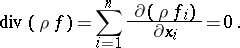In this case the flow has an invariant measure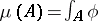, which is given in (local) coordinates by its density(the latter is often called an integral invariant, allowing for some ambiguity of speech).

A Hamiltonian system with (generalized) momenta and coordinates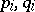,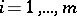, has the relative integral invariantand the absolute integral invariant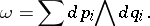This fact may be put at the basis of the definition of a Hamiltonian system and may be used to develop the theory of Hamiltonian systems, since many specific properties of such systems are directly related to these integral invariants (cf. , ). The exterior powers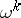(including the volume form) are absolute, while the products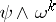are relative integral invariants of any Hamiltonian system. Therefore, they are called universal integral invariants of Hamiltonian systems. Up to a multiplier, all universal integral invariants of Hamiltonian systems can be reduced to the ones indicated (cf. , ).

If (1) has an absolute integral invariantof degree, then for any-dimensional smooth chain(e.g., for a smooth-dimensional manifold),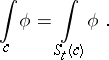(2)

If (1) has a relative integral invariant, then (2) holds, generally speaking, only when the chain is the boundary of a chain of dimension. Sometimes relative integral invariants are defined by the stronger condition that (2) holds for all cycles. Initially, integral invariants were defined by H. Poincaré (cf. , ) as integrals of the type above that remain invariant under the action of the flow on the domain of integration.

All that has been said can easily be generalized to non-autonomous systems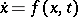. The modification given by E. Cartan (cf. ) appears to be most essential. It involves the transition (even in the autonomous case) to an extended phase space (one adds time to the ordinary phase coordinates), in which the integral curves (cf. Integral curve) of the system of differential equations considered form a certain family of lines (a congruence, cf. Congruence of lines). Cartan requires that the integral of a formover a chain(or over a cycle, if one discusses a relative integral invariant) remains invariant if each pointmoves along the integral curve passing through this point; different points may move in different ways as long as this gives a smooth deformation of. (E.g., in the new sense it is notthat is a relative integral invariant of Hamiltonian systems, but the extremely useful Cartan–Poincaré integral invariant, whereis the Hamiltonian, cf. .) A related definition is given in .

How to Cite This Entry:
Integral invariant. Encyclopedia of Mathematics. URL: http://encyclopediaofmath.org/index.php?title=Integral_invariant&oldid=12165
This article was adapted from an original article by D.V. Anosov (originator), which appeared in Encyclopedia of Mathematics - ISBN 1402006098. See original article